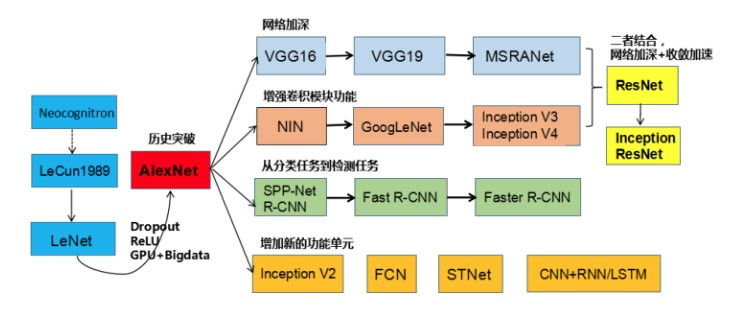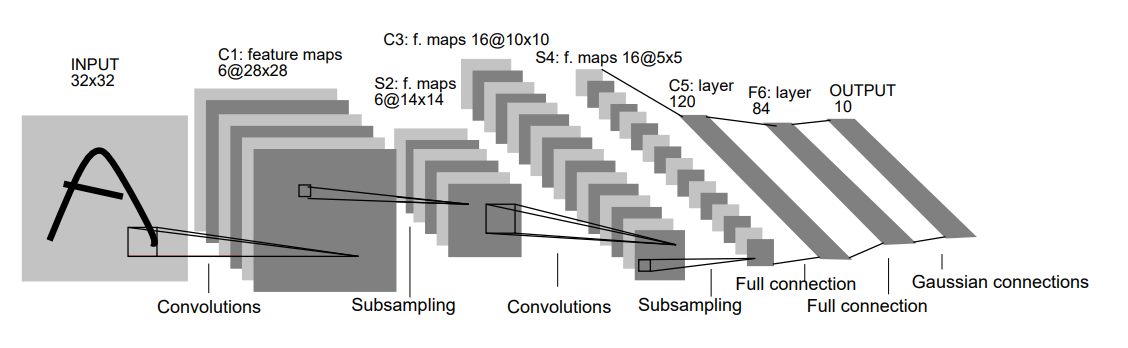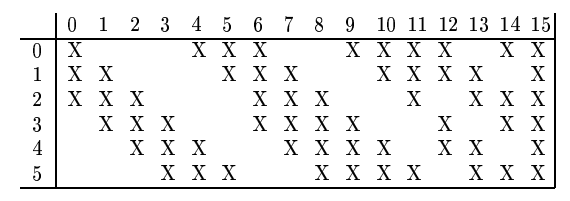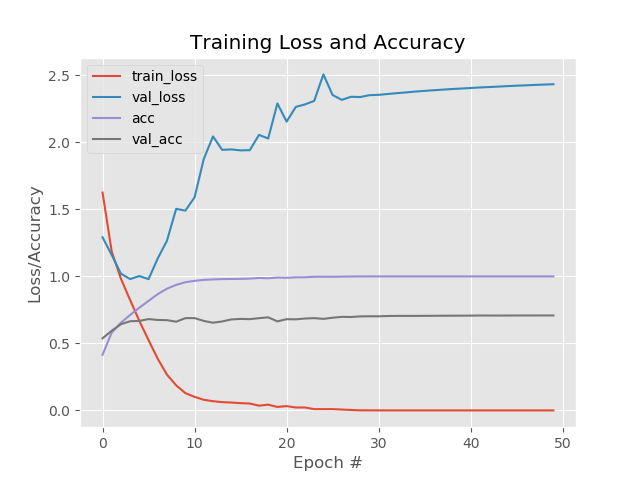# 卷积神经网络之LeNet

• A+• LeNet
• AlexNet
• VGG
• GoogLeNet
• ResNet

• LeNet 网络结构
• LeNet 论文
• LeNet keras实现，并应用CIFAR10进行测试

## LeNet的贡献

LeNet-5可谓是第一个卷积神经网络，并且在手写数字识别上取得了很好的效果。

1. 图像数据量较大，单独像素独立输入神经元中，需要很大的网络结构，训练参数过多
2. 图像的形变，形变引起的图像特征位置变化的问题
3. 图像的局部相关性。

• 局部感受野
• 权值共享
• 下采样(池化)

## LeNet网络结构

1998年的诞生的LeNet(LeCun et al. Gradient-Based Learning Applied to Document Recognition)可谓是现在各种卷积神经网络的始祖了，其网络结构虽然只有5层，却包含了卷积神经网络的基本组件（卷积层，池化层，全连接层）• 输入层 INPUT
输入$$32\times32\times1$$的图像
• 第一个卷积层 CONV1
使用6个尺寸为$$5 \times 5$$的滤波器，在卷积的过程中不做边缘填充，步长为$$stride=1$$。单个核的卷积输出大小为$$(32 - 5 + 1) \times (32 - 5 + 1) = 28 \times 28$$。由于有6个卷积核，所以整个卷积层输出得到为Feature Map为 $$28 \times 28 \times 6$$。该层的参数
• 滤波器的核： $$(5 \times 5 + 1) \times 6 = 156$$，每个滤波器除了$$5 \times 5$$的核以外，还有一个偏置。
• 神经元的个数：$$28 \times 28 \times 6$$在卷积层中，卷积核每移动一步产生一个神经元的输出，也就相当于一个神经元。卷积的过程中，不做边缘填充，对于$$32 \times 32$$的输入，一个$$5\times5$$的卷积核产生$$28 \times 28$$个输出，共有6个卷积核，有$$28 \times 28 \times 6$$个神经元。
• 总共的连接数： $$(5 \times 5 + 1) \times 28 \times 28 \times 6 = 122304$$。在卷积层，每个输出的$$28 \times 28$$的Feature Map，都和一个$$5 \times 5$$卷积核相连。每个卷积核都有一个偏置。
• 参数个数：按照神经网络的全连接设计，每个像素间都有连接的话，输入层和卷积层之间就要有122304个参数。但是在卷积神经网络中，引入了权值共享，就大大的减少了卷积层的参数的个数。对$$32\times32$$的输入，使用$$5\times5$$的核进行卷积，步长为1，卷积核每移动一步，就得到一个输出，也就需要一个权值参数(有一个连接)，这就需要$$(5 \times 5 + 1) \times 28 \times 28$$个参数。引入权值共享，也就是一个卷积核在卷积的产生输出的过程中，每移动一步产生输出，都使用相同的权值参数，这时候参数的个数为$$(5 \times 5 + 1) \times 1$$。共有6个卷积核，$$(5 \times 5 + 1) \times 6 = 156$$个参数。

设输入尺寸为$$W \times W$$，卷积核尺寸为$$F \times F$$，卷积步长为$$S$$，填充宽度为$$P$$，则卷积后输出的尺寸为$$(W - F + 2P) / S + 1$$

• 池化层 POOL1
池化层用于降采样，其输入是上一卷积层的输出为$$28 \times 28 \times 6$$。在本层采用尺寸$$2 \times 2$$的池化单元，池化时的沿长和宽的步长均为2。 池化后的输出为$$(28 - 2) / 2 + 1 = 14$$。深度为6，则池化的输出为$$14 \times 14 \times 6$$
• 连接个数： $$(2 \times 2 + 1 ) \times 14 \times14 \times 6 = 5880$$
• 参数个数：和卷积层类似，每个池化单元进行池化的时候使用相同的权值，则训练参数为$(1 + 1) \times 6 = 12$$。 其中，一个是池化单元的权值$$w$$,另一个是偏置参数。 > 池化层输入尺寸为$$W \times W$$，池化尺寸为$$F \times F$$，池化步长为$$S$$，则池化后的输出尺寸为$$(W - F)/S + 1$
• 第二个卷积层 CONV2
该层的输入为$$14 \times 14 \times 6$$,使用16个核为$$5\times5$$,步长为1。则其输出尺寸为$$14 - 5 + 1 = 10$$。整个层的输出为$$10 \times 10 \times 16$$。 这里要注意的本层的输入有6个Feature Map，而输出有16个Feature Map。 输入的FeatureMap和输出的FeatureMap之间并不是全连接的，而是局部连接的，具体规则如下：- 参数个数： 由于权值共享，每个卷积核产生的输出共享同一个权值。例如，对于和3个Feature Map相连接的输出的FeatureMap，在卷积的时候，使用3个不同的卷积核分别对三个输入的FeatureMap进行卷积，然后将卷积的结果进行求和，所以其权值参数的个数是$$5 \times 5 \times + 1$$。所以，总的参数个数是$$6 \times (5 \times 5 \times 3 + 1) + 9 \times ( 5 \times 5 \times 4 + 1) + 1 \times (5 \times 5 \times 6 + 1) = 1516$$
- 连接个数：输出的Feature Map的尺寸是$$10 \times 10$$，则连接个数是： $$1516 \times 10 \times = 151600$$

• 第二个池化层 POOL2
输入是$$10 \times 10 \times 16$$，池化参数和第一个池化层一样采用尺寸$$2 \times 2$$的池化单元，池化时的沿长和宽的步长均为2，其输出为$$5 \times 5 \times 16$$，参数个数是$$(1 + 1) \times 16 = 32$$，连接数$$(2 \times 2 + 1) \times 5 \times 5 \times 16 = 2000$$

• 第三个卷积层 CONV3
120个$$5\times5$$的卷积核，输入的是$$5 \times 5 \times 16$$,输入的Feature Map和输出的Feature Map是全连接，输出的尺寸为$$1\times1 \times 120$$(卷积核和输入尺寸相同，且没有边缘填充)。其卷积核的参数是$$5\times5 \times 16 + 1$$。 而输出的120个神经元每个都和上一层的相连，则连接和参数的个数相同为$$(5\times5 \times 16 + 1) \times 120 = 48120$$

• 全连接层
输入是120维的向量，本层有84个神经元，参数个数是$$(120 + 1) \times 84 = 10164$$

• 输出层
全连接层，10个神经元，代表10个不同的数字。参数/连接个数为$$84 \times 10 = 840$$

## LeNet论文

LeNet是在1998年提出的，用于手写体数字的识别， 首次提出了卷积神经网络的基本组成：卷积层，池化层和全连接层以及权值共享，感受野等概念。 虽然时间比较久i，但是作为卷积神经网络的开山之作，还是值得入门者研读一番的。

• 传统的机器学习进行图像分类，需要手工的设计特征提取器从图像集中提取特征，然后输入到机器学习算法中进行学习。
• 使用梯度下降的方法训练的多层神经网络，可以直接从大量的数据集中学习到复杂的，高维度以及非线性的特征，这些特征远比人工设计的特征要好很多。
• 最后，可以在神经网络的全连接层直接利用前面网络层提取到的特征进行分类，不用像传统的机器学习分类那样，分成两个步，而是进行end-to-end的学习。

• 训练难。图像的数据由一个个的像素组成，如果将每个像素都输入到一个的单独的神经元中，那神经网络的尺寸，权值参数将是非常的大的。这么多的参数，需要消耗非常多的计算资源，以及需要非常大的数据集。
• 无法应对图像内容位置的变化。对于手写体的数字图像，其每个字符的大小，倾斜度，书写的位置都会导致特征位置的变化。如果将每个像素输入到一个单独的神经元中，则需要大量的不同位置的训练数据输入到神经元中。
• 忽略了图像的局部相关性。 图像的相邻像素有很强的相关性。单独一个像素输入到神经元中则丢失了这种相关性。

• 利用卷积核对输入进行卷积后输入到神经元中，来保持图像局部相关性
• 使用局部感受野，权值共享，池化（下采样）来实现图像的平移，缩放和形变的不变性。

## Keras实现

_________________________________________________________________
Layer (type)                 Output Shape              Param #
=================================================================
conv2d_1 (Conv2D)            (None, 32, 32, 20)        1520
_________________________________________________________________
activation_1 (Activation)    (None, 32, 32, 20)        0
_________________________________________________________________
max_pooling2d_1 (MaxPooling2 (None, 16, 16, 20)        0
_________________________________________________________________
conv2d_2 (Conv2D)            (None, 16, 16, 50)        25050
_________________________________________________________________
activation_2 (Activation)    (None, 16, 16, 50)        0
_________________________________________________________________
max_pooling2d_2 (MaxPooling2 (None, 8, 8, 50)          0
_________________________________________________________________
flatten_1 (Flatten)          (None, 3200)              0
_________________________________________________________________
dense_1 (Dense)              (None, 500)               1600500
_________________________________________________________________
activation_3 (Activation)    (None, 500)               0
_________________________________________________________________
dense_2 (Dense)              (None, 10)                5010
_________________________________________________________________
activation_4 (Activation)    (None, 10)                0
=================================================================
Total params: 1,632,080
Trainable params: 1,632,080
Non-trainable params: 0
_________________________________________________________________

# -*- coding:utf-8 -*-

from keras.models import Sequential
from keras.layers.convolutional import Conv2D
from keras.layers.convolutional import MaxPooling2D
from keras.layers.core import Activation
from keras.layers.core import Flatten
from keras.layers.core import Dense
from keras import backend as K

class LeNet:
@staticmethod
def build(width,height,depth,classes):
model = Sequential()
inputShape = (height,width,depth)

if K.image_data_format() == "channels_first":
inputShape = (depth,height,width)

# first set of CONV => RELU => POOL
model.add(Conv2D(20,(5,5),padding="same",input_shape=inputShape))
model.add(Activation("relu"))
model.add(MaxPooling2D(pool_size=(2,2),strides=(2,2)))

# second set of CONV => RELU => POOL_layers
model.add(Conv2D(50,(5,5),padding="same"))
model.add(Activation("relu"))
model.add(MaxPooling2D(pool_size=(2,2),strides=(2,2)))

# set of FC => RELU layers
model.add(Flatten())
model.add(Dense(500))
model.add(Activation("relu"))

# softmax classifier
model.add(Dense(classes))
model.add(Activation("softmax"))

return model

print("[INFO] loading CIFAR-10 data...")
((trainX,trainY),(testX,testY)) = cifar10.load_data()
trainX = trainX.astype("float")  /  255.0
testX = testX.astype("float")  /  255.0

lb =  LabelBinarizer()
trainY = lb.fit_transform(trainY)
testY = lb.fit_transform(testY)

print("[INFO] compiling model...")
opt =  SGD(lr=0.05)
model = lenet.LeNet.build(width=width,height=height,depth=depth,classes=classes)
model.compile(loss="categorical_crossentropy",optimizer=opt,metrics=["accuracy"])

# train
print("[INFO] training network...")
H = model.fit(trainX,trainY,validation_data=(testX,testY),batch_size=32,epochs=epochs,verbose=1)

# evaluate the network
print("[INFO] evaluating networking...")
predictions = model.predict(testX,batch_size=32)
print(classification_report(testY.argmax(axis=1),predictions.argmax(axis=1),
target_names=labelNames))